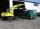# Brick

Isosceles scale has on one side all brick and second weight 1 kg and 1/4 of brick. The balance is in equilibrium.

What is the weight of a brick?

Result

m =  1.3 kg

#### Solution:Leave us a comment of example and its solution (i.e. if it is still somewhat unclear...):Be the first to comment!#### To solve this example are needed these knowledge from mathematics:

Need help calculate sum, simplify or multiply fractions? Try our fraction calculator. Do you have a linear equation or system of equations and looking for its solution? Or do you have quadratic equation?

## Next similar examples:

1. Brick weightHalf the weight of a brick plus 20 pounds is equal to 1/3 the weight of the brick plus 30 pounds. How much does the brick weigh?
2. Coal storageThe coal storage distribute received coal shipment within three days. The first day distribute third of the shipments, the second day of two-fifths of the rest and the third day 300 tons of coal. How many tons of coal distributed first and second day?
3. Equation 15Solve equation with variables on both sides:
4. ClassroomOne-eighth of 9th class was interested in studying at a grammar school, at a business academy one sixth, at secondary vocational schools quarter, to SOU one third and the remaining three students were interested in the school of art direction. How many st
5. Fraction and a decimalWrite as a fraction and a decimal. One and two plus three and five hundredths
6. ZdeněkZdeněk picked up 15 l of water from a 100-liter full-water barrel. Write a fraction of what part of Zdeněk's water he picked.
7. Mixed2improperWrite the mixed number as an improper fraction. 166 2/3
8. Lengths of the poolMiguel swam 6 lengths of the pool. Mat swam 3 times as far as Miguel. Lionel swam 1/3 as far as Miguel. How many lengths did mat swim?
9. In fractionsAn ant climbs 2/5 of the pole on the first hour and climbs 1/4 of the pole on the next hour. What part of the pole does the ant climb in two hours?
10. Equation 20In given equation: 8/9-4/5=2/9+x, find x
11. Forest nurseryIn the forest nursery after winter, they found that 1/10 stems died out of them. For them, they land 193 new spruces. How many spruces are in the forest nursery?
12. Simple equation 5Solve equation with fractions: X × 3/8 = 1/2
13. Fraction to decimalWrite the fraction 3/22 as a decimal.
14. FlourKim needs 3/4 cup of flour to make 12 cookies. How much flour would she need to make 60 cookies?Mom bake cookies. Rolo took 2/9 of all cookies, Michal 3/9. How many cookies ate Rolo if Michal had 9.Determine the unknown number, which is by 1.5 greater than its fourth.3/5 trees are apples, cherries are 1/3. 5 trees are pear. How many is the total number of trees?